# GSEB Solutions Class 11 Physics Chapter 11 Thermal Properties of Matter

Gujarat Board GSEB Textbook Solutions Class 11 Physics Chapter 11 Thermal Properties of Matter Textbook Questions and Answers.

## Gujarat Board Textbook Solutions Class 11 Physics Chapter 11 Thermal Properties of Matter

### GSEB Class 11 Physics Thermal Properties of Matter Text Book Questions and Answers

Question 1.
The triple point of neon and carbon dioxide are 24.57 K and 216.55 K respectively. Express these temperature on the Celsius and Fahrenheit scales?
Here, Triple point of neon, T1 = 24.57 K
and Triple point of CO2, T2 = 216.55 K
We know that the temperature of a body on Celsius, F and R, and absolute scale are related as:
$$\frac{C-0}{100-0}$$ = $$\frac{F-32}{212-32}$$
= $$\frac{R-0}{80-0}$$ = $$\frac{T-273.15}{100}$$
On Celsius scale: $$\frac{C-0}{100-0}$$ = $$\frac{T-273.15}{100}$$
or C = T – 273.15
For neon, $$\mathrm{t}_{1}^{\circ}{C}$$ = 24.57 – 273.15 = -248.58°C
For CO2, $$\mathrm{t}_{2}^{\circ}{C}$$ = 216.55 – 273.15 = – 56.6°C

On Fahrenheit Scale:
$$\frac{F-32}{180}$$ = $$\frac{T-273.15}{100}$$
For neon F1 = (T1 – 273.15) × $$\frac{9}{5}$$ + 32
= (24.57 – 273.15) × $$\frac{9}{5}$$ + 32
= -248.58 × $$\frac{9}{5}$$ + 32
= -415.44°F

For CO2,
F2 = (T2 – 273.15) × $$\frac{9}{5}$$ + 32
= (216.55 – 273.15) $$\frac{9}{5}$$ + 32
= -56.6 × $$\frac{9}{5}$$ + 32
= -69.88°F.Question 2.
Two absolute scales A and B have triple-points of water defined to be 200 A and 350 B. What is the relation between TA and TB?
Here, triple point of water on absolute scale A = 200 A
and triple point of water on absolute scale B = 350 B
Also we know that triple point of water on absolute scale = 273.16 K
Thus it follows that temperature 200 A and 350 B on absolute scale A are equivalent to temperature 273.16 on absolute scale, i.e. the absolute scales measure the triple point hs 200A and 350B.
∴ Size of one degree of Kelvin scale on absolute scale A = $$\frac{273.16}{200}$$
or 1A = $$\frac{273.16}{200}$$K
and size of one degree of Kelvin scale on absolute scale B = $$\frac{273.16}{350}$$
or 1B = $$\frac{273.16}{350}$$K
or Value of temperature TA on absolute scale = $$\frac{273.16}{200}$$ × TA
and Value of temperature TB on absolute scale = $$\frac{273.16}{350}$$ × TB
As TA and TB are the same temperature.
∴ $$\frac{273.16}{200}$$ × TA = $$\frac{273.16}{350}$$ × TB
or TA = $$\frac{200}{350}$$ × TB
or TA = $$\frac{200}{350}$$TB = $$\frac{4}{7}$$TB.

Question 3.
The electrical resistance in ohms of a certain thermometer varies with temperature according to the approximate law:
R = R0[1 + 5 × 10-3(T – T0)]
The resistance is 101.6 Ω at the triple-point of water, and 165.5 Ω at the normal melting point of lead (600.5 K). What is the temperature when the resistance is 123.4 Ω?
Here, R1 = 101.6 Ω at T1 = 273.16 K
R2 = 165.5 Ω at T2 = 600.5 K
Let R0 is the resitance at T0.
and let R3 = 123.4 Ω at Temp. T3 = ?
Here the eiven law for electrical resistance as a function of temperature is given by:
R = R0[1 + 5 × 10-3(T – T0)] ………………… (i)
∴ 101.6 = R0[1 + 5 × 10-3(273.16 – T0)] ………………… (ii)
and 165.5 = R0[1 + 5 × 10-3(600.5 – T0)] …………………. (iii)
$$\frac{(iii)}{(ii)}$$ gives, $$\frac{165.5}{101.6}$$ = $$\frac{1+5 \times 10^{-3}\left(600.5-T_{0}\right)}{1+5 \times 10^{-3}\left(273.16-T_{0}\right)}$$
or 1 + 5 × 10-3(600.5 – T0) = 1.629[1 + 5 × 10-3(273.16 – T0)]
or 1.629(1 + 1.366 – 0.005 T0) = 1.629[1 + 5 × 10-3(273.16 – T0)]
or 3.854 – 0.008 T0 = 4.003 – 0.005 T0
or 0.003T0 = 0.149
or T0 = -49.67 K
∴ From (ii), R0 = $$\frac{101.6}{1+0.005(273.16+49.67)}$$
= $$\frac{101.6}{2.614}$$ = 38.87 Ω
∴ 123.4 = 38.87 {1 + 0005 [T – (-49.67)]}
or T + 49.67 = ($$\frac{123.4}{38.87}$$ – 1) $$\frac{1}{0.005}$$
or T = 434.94 – 49.67 = 385 K.

Aliter:
R = R0[1 + 5 × 10-3(T – T0)]
Also we know that R1 = R0[1 + ∝(T – T0)]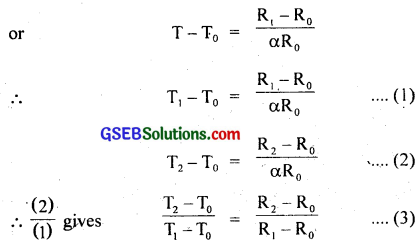Here R0 is resistance at T0
i.e. T0 = 273.16 K, R0 = 101.16Ω, T1 = 600.5 K, R1 = 165.5 Ω, T2 = ?, R2 = 123.4 Ω. …………………….. (4)
∴ From (3) and (4), we get
T2 – T0 = ($$\frac{123.4-101.6}{165.5-101.6}$$) (600.5 – 273.16)
= 384.88 = 385 K.Question 4.
(a) The triple point Of water is a standard fixed point in modern thermometer. Why? What is wrong in taking melting point of ice and the boiling point of water as standard fixed points (as was originally done in Celsius scale)?
(b) There were two fixed points in the original Celsius scale as mentioned above which were assigned the numbers 0° C and 100° C respectively. On the absolute scale one of the fixed points is the triple point of water, which on the Kelvin absolute scale is assigned the number 273.16 K. What is the other fixed point on this (Kelvin) scale.
(c) The absolute temperature (Kelvin scale) T is related to the temperature T on the Celsius scale by tc = T – 273.15. Why do we have 273.15 in this relation and not 273.16?
(d) What is the temperature of triple point of water on an absolute scale whose unit interval size is equal that of the Fahrenheit scale?
(a) This is because the triple point of water is unique condition of temperature and pressure i.e. it occurs only at one particular set of values of pressure and temperature. So it is easily reproducible. Hence it is used as a standard temperature in thermometry. The melting point of ice and boiling point of water are pressure dependent i.e. they change with change in the value of pressure. They are also, very sensitive to the dissolved impurities. Hence these have been discarded as a reference point and triple point of water has been taken as a fixed point.

(b) The OK or absolute zero itself is the other fixed point on the absolute scale of temperature. It corresponds to the temperature when the volume and pressure of a gas will become zero.

(c) Triple point is 0.01°C and not 0°C. Thus to make a Celsius degree equal to Kelvin degree, 273.15 is assigned instead of 273.16. Moreover ice point on Kelvin scale is 273.15K and the corresponding ice point on Celsius scale is 0°C which is clear from the relation i.e. tc = T – 273.15. If we have 273.16 instead of 273.15 in the above relation, then the ice point on Celsius scale will be – 0.01°C which is not the case.

(d) The unit interval size of Fahrenheit scale is 212 – 32 = 180 divisions. Also we know that the unit interval size of absolute scale is 100.
∴ Triple point of water on an absolute scale having 180 divisions is given by
T = $$\frac{273.16}{100}$$ × 180 = 491.69.

Question 5.
Two ideal gas thermometers A and B use oxygen and hydrogen respectively. The following observations are made: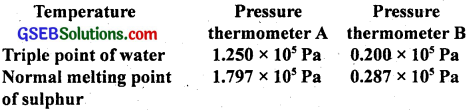(a) What is the absolute temperature of normal melting point of sulphur as read by thermometers A and B?
(b) What do you think is the reason for slightly different answers of thermometers A and B? (The thermometers are not faulty). What further procedure is needed in the experiment to reduce the discrepancy between the two readings?
(a) Let T be the melting point of sulphur. Also we know that triple point of water Ttr = 273.16 K.
For thermometer A, Ptr – 1.250 × 105 Pa, P = 1.797 × 105 Pa, T = ?
Using the relation,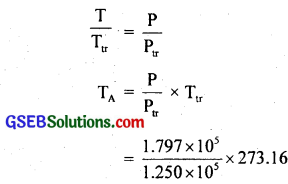= 392.69 K.
For thermometer B, Ptr = 0.200 × 105 Pa, P = 0.287 × 105 Pa
TB = Ttr × $$\frac{P}{P_{t r}}$$
= 273.16 × $$\frac{0.287 \times 10^{5}}{0.200 \times 10^{5}}$$
or TB = 391.98 K.

(b) The value of the melting point of sulphur found from the two thermometers differ slightly due to the reason that in practice, the gases do not behave strictly as perfect gases i.e. gases are not perfectly ideal. To reduce the discrepency, readings should be taken for lower and lowjer pressures and the plot between temperature measured versus absolute pressure of the gas at triple point should be extrapolated to obtain temperature in the limit pressure tends to zero (i.e. P → 0), when the gases approach ideal gas behaviour.Question 6.
A steel tape 1 m long is correctly calibrated for a temperature of 27.0°C. The length of steel rod measured by this tape is found to be 63.0 cm on a hot day when the temperature is. 45.0°C. What is the actual length of the steel rod on that day? What is the length of the same steel rod on a day when temperature is 27.0°C? Coefficient of linear expansion of steel = 1.20 × 10-5K-1?
Steel tape is calibrated at 27°C. So it measures correct length at 27°C i.e. size of 1 cm on steel tape has correct magnitude.
∴ Length of steel rod at 27°C, l0 = 63 cm, α = 1.2 × 10-5K-1.
If ∆l be the increase in the length of steel rod or steel tape when temperature rises from 27°C to 45°C,
Then ∆l = α0∆t
= 1.2 × 10-5 × 63 × 18 cm
(∵∆T = 45 – 27 = 18°C)
= 0.0136 cm.
∴ The actual length of the steel rod at 45°C
= l0 + ∆l
= 63 + 0.0136
= 63.0136 cm
The data is correct only upto three significant figures. The actual length of the rod is 63.0 cm, but the change in length of the rod is 0.0136 cm. On a day when temperature is 27°C, the size of 1 cm mark on the steel tape will be exactly 1 cm (due to calibration of steel tape cm 27°C)
∴ length of rod at 27°C = 63.0 × 1 = 63.0 cm.

Aliter:
The length of steel tape of 27°C is 100 cm.
∴ l0 = 100 cm
T = 27°C.
∴ l’ = length of 45°C = l0 + αl0 ∆T
= 100 + 1.2 × 10-5 × 100 × (45 – 27)
= 100.0216 cm
∴ length of 63 cm measured by this tape at 45°C will actually be
= $$\frac{100.0216}{100}$$ × 63 = 63.0136 cm.

Question 7.
A large steel wheel is to be fitted on a shaft of the same material. At 27°C, the outer diameter of the shaft is 8.70 cm and the diameter of the central hole in the wheel is 8.69 cm. The shaft is cooled using ‘dry ice’. At what temperature of the shaft does the wheel slip on the shaft? Assume the coefficient of linear expansion of the steel to be constant over the required temperature range?
αsteel = 1.20 × 10-5K-1
l1 = 8.70 cm
l2 = 8.69 cm
T1 = 20°C = 273 + 27 = 300 K
T2 = ?
When the shaft cooled to temp. T2 such that l2 = 8.69 cm, the wheel can slip on the shaft.
∴ From (1) and (2), we get
8.69 = 8.70[1 + 1.20 × 10-5(T2 – 300)]
or T2 = 300 – 95.78 = 204.22 K
= 204.22 – 273.15 = -68.93°C
or T2 = -69°C.Question 8.
A hole is drilled in a copper sheet. The diameter of the hole is 4.24 cm at 27.0°C. What is the change in the diameter of the hole when the sheet is heated to 227°C? Coefficient of linear expansion of copper = 1.70 × 10-5°C-1?
Here, t1 = 27°C
t2 = 227°C
Coefficient of linear expansion a for copper = 1.70 × 10-5°C-1
∴ ∆t = t2 – t1 = 227 – 27 = 200.0°C
Diameter of hole at 27°C, d1 = 4.24 cm.
Let diameter of hole at 227°C be d2
∴ ∆d = d2 – d1 = ?
If β be the coefficient of superficial expansion of copper,
then β = 2α = 2 × 1.7 × 10-5 = 3.4 × 10-5°C-1
If S1 = $$\frac{\pi \mathrm{d}_{1}^{2}}{4}$$ = $$\frac{π}{4}$$ × (4.24)2 = 4.494πcm2
∴ S2 = S1(1 + β ∆t)
= 4.494 π(1 + 3.40 × 10-5 × 200)
or S2 = 4.494 π × 1.0068 = 4.525 πcm3
or $$\frac{\pi \mathrm{d}_{2}^{2}}{4}$$ = 4.525 π
or d2 = $$\sqrt {4.525×4}$$ = 4.2544 cm
∴ ∆d = d2 – d1 = 4.2544 – 4.24
= 0.0144 cm
or ∆d = 1.44 × 10-2 cm.

Question 9.
A brass wire 1.8 m long at 27°C is held taut with little tension between two rigid supports. If the wire is cooled to a temperature of -39°C, what is the tension developed in the wire, if its diameter is 2.0 mm? Coefficient of linear expansion of brass = 2.0 × 10-5K-1. Young’s modulus of brass – 0.91 × 1011 Pa?
Here, l1 = 1.8 m, t1 = 27°C, t2 = -39°C
∴ ∆t = t2 – t1 = – 39 – 27 = – 66°C
l2 = length at t2°C
For brass, α = 2 × 10-50K-1
∴ Y = 0.91 × 1011 Pa
diameter of wire, d – 2.0 mm = 2.0 × 10-3 m
If a be the area of cross-section of the wire, then
a = $$\frac{\pi \mathrm{d}_{2}^{2}}{4}$$ = $$\frac{π}{4}$$ × (2.0 × 10-3)2
= 3.142 × 10-6m2.
If F be the tension developed in the wire, then using the relation
Y = $$\frac{F/a}{∆l/L}$$, we get
F = $$\frac{\mathrm{Ya} \Delta l}{l_{\mathrm{i}}}$$ ……………………. (1)
Now ∆l = l1α∆t = 1.8 × 2 × 10-5 × (-66)
= – 0.00237 m = – 0.0024 m
– ve sign shows that the length decreases.
Putting values of Y, a, ∆l and l1, in equation (1), we get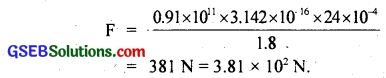Question 10.
A brass rod of length 50 cm and diameter 3.0 mm is joined to a steel rod of the same length and diameter. What is the change in length of the combined rod at 250°C, if the original lengths are at 40°C? Is there a ‘thermal stress’ developed at the junction? The ends of the rod are free to expand (coefficient of linear expansion of brass = 2.0 × 10-5K-1, steel = 1.2 × 10-5K-1.)
For brass rod, α = 2.0 × 10-5K-1, l1 = 50 cm, t1 = 40°C, t2 = 250°C.
∴ ∆t = t2 – t1 = 250 – 40 = 210°C.
If l2 be its length at t2°C, then
l2 = l1(1 + α∆t)
= 50(1 + 2 × 10-5 × 210)
= 50.21 cm
∴ ∆l brass = l2 – l1 = 50.21 – 50 = 0.21 cm

For steel rod:
t’1 = 40°C, t’2 = 250°C, α = 1.2 × 10-5K-1, l’1 = 50.0 cm, ∆t’ = t’2 – t’1 = 250 – 40 = 210°C.
If l’2 be the length of the steel rod at 250°C, then
l’2 = l’1(1 + α ∆t’)
= 50(1 + 1.2 × 10-5 × 210)
= 50.126 cm.
∴ ∆lsteel = l’2 – l’1 = 50.126 – 50
= 0.126cm
= 0.13 cm
∴ the length of the combined rod at 250°C = l2 + l’2
= 50.21 + 50.126
= 100.336 cm
and length of the combined rod at 40°C = l1 + l’1
= 50 + 50
= 100 cm
∴ Total change in length of the combined rod = 100.336 – 100
= 0.336 cm
= 0.34 cm.
No ‘thermal stress’ is developed at the junction since the rods freely expand.

Question 11.
The coefficient of volume expansion of glycerine is 49 × 10-5K-1. What is the fractional change in its density for a 30°C rise in temperature?
Here, γ = 49 × 10-5K-1
rise in temperature, ∆t = 30°C
Let V0 be the initial volume of glycerine i.e. at 0°C (dry)
If V1 be its volume at 30°C
Then Vt = V0(1 + γ∆t)
= V0 (1 + 49 × 10-5 × 30)
= V0(1 + 0.01470) = 1.01470V0
or $$\frac{\mathrm{V}_{0}}{\mathrm{~V}_{\mathrm{t}}}$$ = $$\frac{1}{1.01470}$$ ………………….. (i)
Let ρ0 and ρt be the initial and final densities of the glycerine,
Then initial density, ρ0 = $$\frac{\mathrm{m}}{\mathrm{V}_{0}}$$
and Final density, ρt = $$\frac{\mathrm{m}}{\mathrm{V}_{t}}$$ where m = mass of glycerine.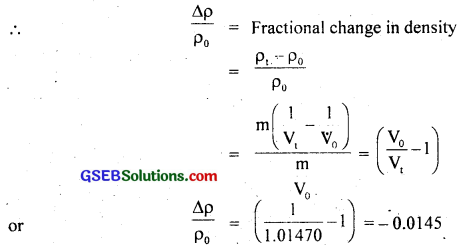Here negative sign shows that the density decreases with the rise in temperature.
∴ $$\frac{\Delta \rho}{\rho_{0}}$$ = 0.0145 = 1.45 × 10-2
= 1.5 × 10-2.Question 12.
A 10 kW drilling machine is used to drill a bore in a small aluminium block of mass 8.0 kg. How much is the rise in temperature of the block in 2.5 minutes, assuming 50% of the power is used up in heating the machine itself or lost to the surroundings. Specific heat of aluminium = 0.91 Jg-1K-1?
Here, power of the machine
P = 10kW= 10 × 103W
time for which the machine is used,
t = 2.5 minute = 2.5 × 60s
= 150s.
mass of machine, M = 8 kg
Specific heat of aluminium, C = 0.91 Jg1K-1
If Q be the energy used up in drilling the bone, then
Q = P × t
= 104 × 150
= 15 × 105J
It is given that 50% of energy is lost to the surroundings
∴ If Q’ = Energy transferred to the aluminium block, then
Q’ = 50% of Q = $$\frac{50}{100}$$ × 15 × 105
Let ∆t°C be the rise in temperature of the block,
∴ Q’ = m C ∆t
or ∆t = $$\frac{Q’}{mC}$$ = $$\frac{75 \times 10^{4}}{8 \times 0.91 \times 10^{3}}$$ = 103°C

Question 13.
A copper block of mass 2.5 kg is heated in furnace to a temperature of 500°C and then placed on a large ice block. What in the maximum amount of ice that can melt? (Specific heat of copper = 0.39 Jg-1K-1; heat of fusion of water = 335 Jg-1)
Here, mass of copper block, m, = 2.5 kg
Specific heat of copper, C = 0.39 Jg-1K-1 – 0.39 × 103 J kg-1K-1
Temperature of furnace, ∆θ = 500°C
Latent heat of fusion, L = 335 Jg-1
= 335 × 103 J Kg-1
If Q be the heat absorbed by the copper block, then
Q = m1C∆θ ………………………. (i)
Let m2(kg) be the mass of ice melted when copper block is placed on it,
∴ Q = m2L ……………………… (ii)
∴ from (i) and (ii), we get
m1C∆θ = m2L
or m2 = $$\frac{\mathrm{m}_{1} \mathrm{C} \Delta \theta}{\mathrm{L}}$$
= $$\frac{2.5 \times 0.39 \times 10^{3} \times 500}{335 \times 10^{3}}$$
= 1.455 kg
= 1.5 kg.Question 14.
In an experiment on the specific heat of a metal a 0.20 kg block of the metal at 150°C is dropped in a copper calorimeter (of water equivalent 0.025 kg) containing 150 cm3 of at 27°C. The final temperature is 40°C. Compute the specific heat of the metal. If beat losses to the surrounding are not negligible is your answer greater or smaller than the actual value for specific heat of the metal?
Here, mass of metal block. m1 = 0.20 kg
temperature of metal block, θ1 = 150°C
temperature of calorimeter and water, θ2 = 27°C
final temperature of the misture. θ = 40°C
Volume of waler in the calorimeter, V = 150 cm3 = 150 × 10-6m3
∴ mass of water in the calorimeter, m2 = Vρ = 150 × 10-6 × 10+3kg
Water equivalent of calorimeter W = 0.025 kg = 25 × 10-3 kg
Let C1 (J kg-1 K-1) be the specific heat of metal block.
∴ Fall in temperature of metal block, ∆θ = θ1 – θ = 150 – 40 = 110°C
Increase in the temperature of water ∆θ = θ – θ2 = 40 – 27 = 13°C
If Q1 = m1C1∆θ
= 0.20 × C1 × 110 = 22C1
Also Let C2 = specific heat of water = 4.2 × 103 J kg-1 K-1
If Q2 be the heat gained by the water and the calorimeter, then
Q2 = (m2 + W)C2∆θ’
= (150 × 10-3 + 25 × 10-3)C2 × 13
= 175 × 10-3C2 × 13
= 175 × 10-3 × 4.2 × 103 × 13
∴ According to the principle of Calorimetry,
Q1 = Q2
or 22C1 = 175 × 4.2 × 13
or C1 = $$\frac{175×4.2×13}{22}$$ = 434 J kg-1 K-1
= $$\frac{434}{1000}$$ J kg-1 K-1 = 0.434 J g-1 K-1
1f heat is lost to the surroundings C will be smaller than the actual value.

Question 15.
Given below are observations on molar specific heats at room temperatures of some common gases?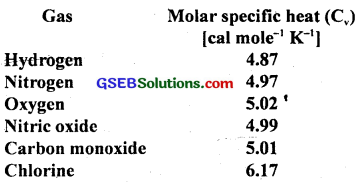The measured molar specific heats of these gases are markedly different from thosefor monoatomic gases (typically molar specific heat of a monoatomic gas is 2.92 cal/mol K). Explain this difference. What can you infer from the somewhat larger (than the rest) value for chlorine?
Since all the given gases are diatomic and have other degrees of freedom i.e. haye other modes of motion (i.e. rotational and vibrational) possible besides the translational degrees of freedom. To raise the temperature of the gas by a certain amount, heat is to be supplied to increase the average energy of all the modes. Consequently, molar specific heat of diatomic gases is more than that of monoatomic gases. It can be shown that if only rotational modes of motion are considered, therfiolar specific heat of diatomic gases is nearly $$\frac{5}{2}$$ R = 2.5 × 1.985 = 4,962 Cal mol-1 K-1 which agrees with the observations for all the gases listed in the table; except chlorine. The higher value of molar specific heat of chlorine indicates that besides translational rotational modes, vibrational modes are also present in chlorine at room temperature.Question 16.
Answer the following questions based on the P – T phase diagram of CO2 as shown in fig. here?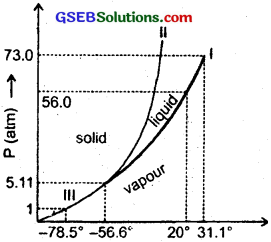(a) At what temperature and pressure can the solid, liquid and vapour phases of CO2 co-exist in equilibrium?
(b) What is the effect of decrease of pressure on the fusion and boiling point of CO2?
(c) What are the critical temperature and pressure for CO2? What is their significance?
(d) Is CO2 solid, liquid or gas at:

• – 70°C under 1 atm,
• – 60°C under 10 atm,
• 15°C under 56 atm?

(a) The solid, liquid and vapour phases of CO2 can exist in equilibrium at its triple point O corresponding to which
and Ptr = 5.11 atm
and Ttr = – 56.6°C

(b) From the vapourisation curve (I) and the fusion curve (II), it follows that both the boiling and fusion point of CO2 decrease with decrease of pressure.

(c) For CO2, Pc = 73.0 atm and Tc = 73.1°C. Above this temperature, CO2 will not liquify even if compressed to high pressure.

(d)

• CO2 is vapour at – 70°C under 1 atm as this point lies in vapour region.
• CO2 is solid at – 60°C under 10 atm as this point lies in solid region.
• CO2 is liquid at 15°C under 56 atm as this point lies in liquid region.

Question 17.
Answer the following questions based on the P – T phase diagram of CO2 as shown in fig. of Q. 11.16.
(a) CO2 at 1 atm pressure and temperature – 60°C is compressed isothermally. Does it go through a liquid phase?
(b) What happens when CO2 at 4 atm pressure is cooled from room temperature at constant pressure?
(c) Describe qualitatively the changes in a given mass of solid CO2 at 10 atm pressure and temperature – 65°C as it is heated up to room temperature at constant pressure.
(d) CO2 is heated to a temperature 70°C and compressed isothermally. What changes in its properties do you expect to observe?
(a) No. CO2 at 1 atm pressure and – 60°C is vapour. If it is compressed isothermally i.e. when pressure is increased without changing the temperature, it will go to solid phase directly without going through the liquid phase.

(b) CO2 at 4 atm pressure and at room temperature (say 25°C) is vapour. If it is cooled at constant pressure, it will again condense to solid without going through liquid phase. (The horizontal line through the initial point intersects only the sublimation curve III).

(c) CO2 at 10 atm pressure and at – 60°C is solid. As CQ2 is heated at constant pressure, it will go to liquid base and then to the vapour phase. It is because, the horizontal line through the initial point intersects both the fusion and vapourisation curves. The fusion and boiling points can be known from the points, where the horizontal line at P – T diagram at 10 atm (initial point) intersects the respective curves.

(d) It will not exhibit any clear phase transition to the liquid phase. However, CO2 gas will depart more and more from the ideal gas behaviour as its pressure increases.Question 18.
A child running a temperature of 101°F is given an antipyrin (i.e. medicine that lowers fever) which causes an increase in the rate of evaporation of sweat from his body. If the fever is thought down to 98°F in 20 min., what is the average rate of extra evaporation caused by the drug. Assume the evaporation mechanism to be the only way by which heat is lost. The mass of child is 30 kg. The specific heat of the human body is approximately the same as that of water and latent heat of evaporation of water at that temperature is about 580 cal g-1.
Here, mass of the child, m = 30 kg
fall in temperature, ∆T = T1 – T2 = 101°F – 98°F
= 3°F
= 3 × $$\frac{5}{9}$$°F
or ∆T = $$\frac{5}{3}$$°C
Specific heat of human body (sp. heat of water)
C = 4.2 × 103 J kg-1C-1
Latent heat of vapourisation = 580 cal g-1
= 5 80 × 4.2 × 103 J kg-1C-1
Let m’ gram of sweat evaporates from the body of the child in 20 min, i.e. t = time taken by sweat to evaporate = 20 min.
If Q be the heat needed, then
Q = m’L
= m × 580 × 4.2 × 103 J …………………. (1)
Also let Q be the heat lost by the child in the form of evaporation of sweat, then
Q = mC∆T
= 30 × 4.2 × 103 × 5 J
= 2.10 × 105 J …………………….. (2)
∴ from (1) and (2), we get
m × 580 × 4.2 × 103 = 2.1 × 105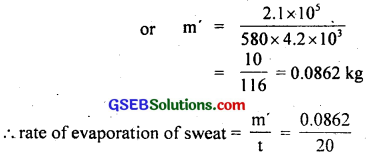= 0.00431 kg min-1
= 4.31 g min-1.

Question 19.
A ‘thermacole’ icebox is a cheap and efficient method for storing small quantities of cooked food in summer in particular. A cubical icebox of side 30 cm has a thickness of 5.0 cm. If 4.0 kg of ice is put in the box, estimate the amount of ice remaining after 6h. The outside temperature is 45°C and coefficient of thermal conductivity of thermacole is 0.01 J s-1m-10K-1. |Heat of fusion of water = 335 × 103J kg-1|
Here,
Area of 6 faces of cube = 6 × 30 × 30 cm2
= 6 × 900 × 104m2
distance, d = 5.0 cm = 5.0 × 10-2m
Total mass of ice, M = 4 kg
Time, t = 6h = 6 × 60 × 60 s
θ1 = temperature outside the box = 45°C
θ1 = temperature inside the box = 0°C
∴ ∆θ = θ1 – θ2 = 45 – 0 = 45°C
Heat of fusion, L = 335 × 103 J kg-1
Coefficient of thermal conductivity of thermacole,
K = 0.01 J S-1 m-10 K-1
Let m = mass (kg) of ice which melts,
∴ heat needed to melt at 0°C, Q = mL ……………………… (1)
Also Q = KA $$\frac{∆θ}{d}$$ t
∴ from (1) and (2), we get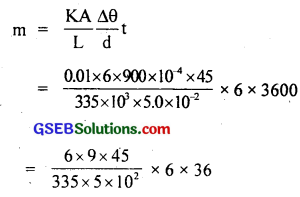= 0.313 kg
∴ Mass of ice left in the box = M – m
= 4.0 – 0.313
= 3.687 kg
= 3.7 kg.Question 20.
A brass boiler has a base area 0.15 m2 and thickness 1.0 cm. It boils jvater at the rate of 6.0 kg/min when placed on a gas stove. Estimate the temperature of the part of the flame in contact with the boiler. Thermal conductivity of brass = 109 J s-1 m-1 K-1. (Heat of vaporization of water = 2256 × 103 J kg-1)
Here, K = 109 Js-1m-1K-1
A = 0.15 m2
d = 1.0 cm = 10-2m
θ2 = 100°C
Let θ1 = temperature of the part of the boiler in contact with the stove.
If Q be the amount of heat flowing per second through the base of the bolier, then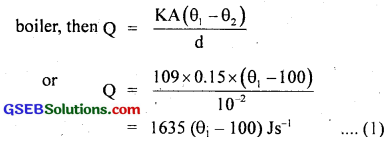Also heat of vaporisation of water,
L = 2256 × 103 Jkg-1
Rate of boiling of water in the boiler,
M = 6.0 kg min-1 = $$\frac{6.0}{60}$$ = 0.1 kg-1s
∴ Heat received by water per second, Q = ML
or Q = 0.1 × 2256 × 103 Js-1 …………………… (2)
∴ From (1) and (2), we get
1635 (θ1 – 100) = 2256 × 102
or θ1 – 100 = $$\frac{2256×100}{1635}$$ = 138
θ1 = 100 + 138 = 238°C

Question 21.
Explain why:
(a) a body with large reflectivity is a poor emitter.
(b) a brass tumbler feels much colder than wooden tray on a chilly day.
(c) an optical pyrfp|eter (for measuring high temperatures) calibrated for Di ideal black body radiation gives too low a value for the temperature of a red hot iron piece in the open, but gives a correct value for the temperature when the same piece is in the furnace.
(d) the Earth without its atmosphere would be inhospitably cold.
(e) heating systems based on circulation of steam are more efficient in warming a building than those based on circulation of hot water.
(a) We know that a + r + t = 1
where a, r and t are absorbtance, reflectance and transmittance respectively of the surface of the body, t is also called emittance (e). Also according to Kirchhoff’s law e ∝ a that is good absorbers are good emitters and hence poor reflectors and vice-versa i.e. If r is large {i.e. large reflectively) ‘a’ is smaller and hence ‘e’ is smaller i.e. poor emitter.

(b) The thermal conductivity of brass is high i.e. brass is a good conductor of heat. So when a brass tumbler is touched, heat quickly flows from human body to the tumbler. Consequently, the tumber appears colder. On the other hand, wood is a bad conductor of heat. So heat does not flow from the human body to the wooden tray, thus it appears relatively hotter.

(c) Let T be the temperature of the hot iron in the furnace. Thus according to Stefan’s law, heat radiated per second per unit area (E) is given by E = σT4. When the body is placed in open at temperature T0, then the heat radiated/sec/area (E’) is given by E’ = σ(T4 – T04). Clearly E’ < E, so the optical pyrometer gives too low a value for the temperature of a red hot iron piece in open.

(d) Gases are generally insulators. The Earth’s atmosphere acts like an insulating blanket around it and does not allow heat to escape out but reflects it back to the Earth. Had this atmosphere been absent, the Earth would naturally be colder as all its heat would have escaped out.

(e) This is because steam has much higher heat capacity (540 cal g-1) than the heat capacity of water (80 cal g-1) at the same temperature. Thus evidently steam will heat building better than water.Question 22.
A body cools from 80°C to 50°C in 5 minutes. Calculate the time it takes to cool from 60°C to 30°C. The temperature of the surroundings is 20°C?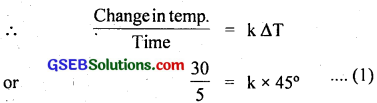∴ $$\frac{30}{t}$$ = k × 25 ……………………. (2)
$$\frac{(1)}{(2)}$$ gives, $$\frac{30/5}{30/t}$$ = $$\frac{k×45}{k×25}$$
or $$\frac{t}{5}$$ = $$\frac{9}{5}$$